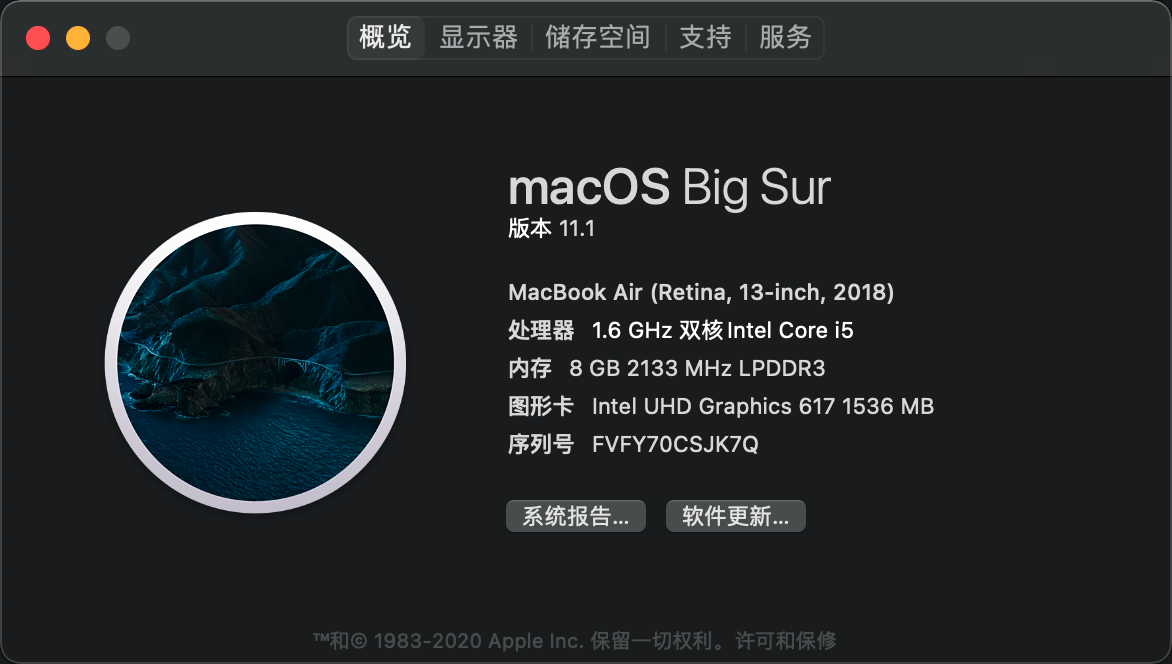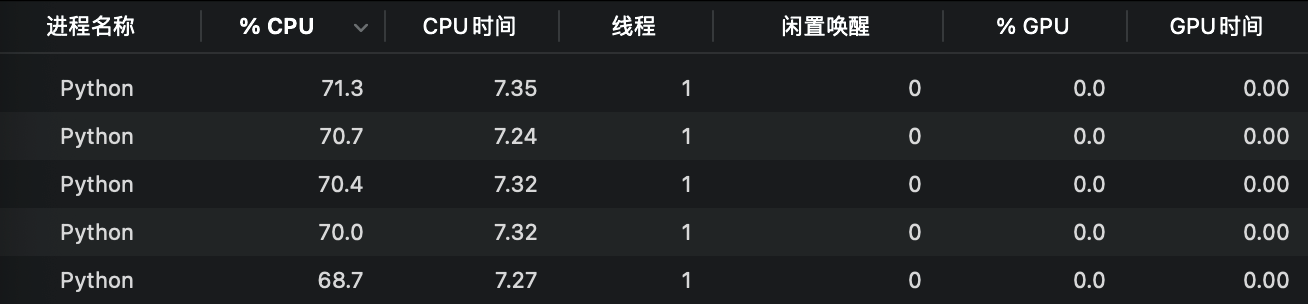# 4.多线程多进程及多线程爬虫开发

1.进程与多进程

2.线程与多线程

3.python中的多线程与多进程

1.使用Process类创建进程

2.使用Pool类实现多进程

3.使用dummy.Pool类实现多线程

# 一、进程与线程

## 3.python中的多线程与多进程

import threading

class threading.Thread(group=None, target=None, name=None, args=(), kwargs={}, *, daemon=None)

group 应该为 None；为了日后扩展 ThreadGroup 类实现而保留。

target 是用于 run() 方法调用的可调用对象。默认是 None，表示不需要调用任何方法。

name 是线程名称。默认情况下，由 "Thread-N" 格式构成一个唯一的名称，其中 N 是小的十进制数。

args 是用于调用目标函数的参数元组。默认是 ()

kwargs 是用于调用目标函数的关键字参数字典。默认是 {}

thread1 = threading.Thread(target = fun)

thread1.start()

使用join()方法控制一个线程执行结束后再执行下一个线程。

join的作用是等待直到线程终结。这会阻塞调用这个方法的线程，直到被调用 join() 的线程终结 -- 不管是正常终结还是抛出未处理异常 -- 或者直到发生超时，超时选项是可选的。

def run():
print('开始执行子线程')
time.sleep(2)
print('子线程执行完毕')

start_time = time.time()
print('主线程开始')
print('主线程执行完毕')
print('花费时间%f'%(time.time()-start_time))

# 输出

import threading
import time

def run():
print('开始执行子线程')
time.sleep(2)
print('子线程执行完毕')

start_time = time.time()
print('主线程开始')
print('主线程执行完毕')
print('花费时间%f'%(time.time()-start_time))

# 输出

import threading
import time

def run():
print('开始制作蛋糕')
time.sleep(2)
print('蛋糕制作完毕')

start_time = time.time()
for i in range(2):
end_time = time.time()
print('single_thread takes %f seconds'%(end_time - start_time))

start_time = time.time()

end_time = time.time()
print('two_thread takes %f seconds'%(end_time - start_time))

if __name__ == '__main__':

# 输出

two_thread takes 2.002863 seconds

def run():
n = 0
print('开始计算')
for i in range(100000000):
n += pow(2*2,3*3)
print('计算完毕')

# 输出

two_thread takes 95.156550 seconds

# 三、多进程库multiprocessing

multiprocessing 是一个用与 threading 模块相似API的支持产生进程的包。 multiprocessing 包同时提供本地和远程并发，使用子进程代替线程，有效避免 Global Interpreter Lock 带来的影响。因此， multiprocessing 模块允许程序员充分利用机器上的多个核心。Unix 和 Windows 上都可以运行。

## 1.使用Process类创建进程

process1 = multiprocessing.Process(target=run)

import multiprocessing
import time
def run():
print('%s进程开始'%(multiprocessing.current_process().name))
time.sleep(2)
print('%s进程结束'%(multiprocessing.current_process().name))

if __name__ == '__main__':
n_cpu = multiprocessing.cpu_count()
print('cpu有%d个核心'%n_cpu)
start_time = time.time()
process1 = multiprocessing.Process(target=run)
process1.start()
process1.join()
print('multiprocess takes %f seconds'%(time.time()-start_time))
# 输出

cpu有4个核心
Process-1进程开始
Process-1进程结束
multiprocess takes 2.201348 seconds

cpu_count用以获取电脑的核心数，作者电脑有2个核心，由于超线程的原因，Python可利用的核心数是真实数量的2倍。current_process用来返回进程这个对象，current_process().name用来返回当前进程的名称。

import multiprocessing
import time
def run():
print('%s进程开始'%(multiprocessing.current_process().name))
result = 0
for num in range(100000):
result +=  pow(99,99)
print('%s进程结束'%(multiprocessing.current_process().name))

if __name__ == '__main__':
n_cpu = multiprocessing.cpu_count()
process_list = []
for n in range(n_cpu):
start_time = time.time()
process = multiprocessing.Process(target=run)
process.start()
process_list.append(process)
for process in process_list:
process.join()
print('multiprocess takes %f seconds'%(time.time()-start_time))

# 输出
Process-1进程开始
Process-2进程开始
Process-3进程开始
Process-4进程开始
Process-1进程结束
Process-2进程结束
Process-4进程结束
Process-3进程结束
multiprocess takes 0.495185 seconds这里有一个细节我们需要注意，在监视器中，一共出现了5个名称为Python的进程，这就是我们使用multiprocessing创建并运行的进程。他们cpu占用的百分比之和小于400%，原因是有4个核心。

import multiprocessing
import time

def run():
n = 0
print('开始计算')
for i in range(100000000):
n += pow(2*2,3*3)
print('计算完毕')

start_time = time.time()
for i in range(2):
end_time = time.time()
print('single_thread takes %f seconds'%(end_time - start_time))

start_time = time.time()

end_time = time.time()
print('two_thread takes %f seconds'%(end_time - start_time))

def two_process():
start_time = time.time()
process1 = multiprocessing.Process(target=run)
process2 = multiprocessing.Process(target=run)

process1.start()
process2.start()

process1.join()
process2.join()
end_time = time.time()
print('two_process takes %f seconds'%(end_time - start_time))

if __name__ == '__main__':
two_process()

# 输出

two_process takes 88.401921 seconds

## 2.使用Pool类实现多进程

multiprocessing提供了一个Pool类来代表进程池对象。Pool可以提供指定数量的进程供用户调用，默认大小是CPU的核数。当有新的请求提交到Pool时，如果池还没有满，那么会创建一个新的进程用来执行该请求；但如果池中的进程数已经达到规定最大值，那么该请求就会等待，指导池中有进程结束，才会创建新的进程来处理她。

import multiprocessing
import os
import time
def run(m):
print(os.getpid(),multiprocessing.current_process().name)
time.sleep(2)

if __name__ == '__main__':
pool = multiprocessing.Pool(processes=2)
for i in range(4):
pool.apply_async(run,args=(i,))
pool.close()
pool.join()

# 输出
60832 SpawnPoolWorker-1
60833 SpawnPoolWorker-2

60833 SpawnPoolWorker-2
60832 SpawnPoolWorker-1
task3 进程结束

## 3.使用dummy.Pool类实现多线程

multiprocessing下面有一个dummy模块，它的下面有一个Pool类，用它可以批量生成线程池，它可以让python的线程使用multiprocessing的各种方法。

from multiprocessing import dummy
import time

def run(m):
time.sleep(2)

if __name__ == '__main__':
pool = dummy.Pool(2)
for i in range(4):
pool.apply_async(run,args=(i,))
pool.close()
pool.join()

# 输出
task3 (id=123145509961728)Thread-1 is ending......

# 四、开发多线程爬虫

import multiprocessing
from multiprocessing import dummy
import time
import requests

def spider_baidu(url):
response = requests.get(url).status_code
print('百度请求成功，状态码%s'%response)

print('单线程开始')
start_time = time.time()
for i in range(10):
spider_baidu(url)
print('单线程结束')
print('单线程花费的时间是%.2f秒'%(time.time()-start_time))

start_time = time.time()
lst = []
for i in range(10):
lst.append(url)
pool = dummy.Pool(4)
pool.map(spider_baidu,lst)
pool.close()
pool.join()
print('多线程花费的时间是%.2f秒'%(time.time()-start_time))

def multi_process(url):
print('%s多进程开始'%multiprocessing.current_process().name)
start_time = time.time()
lst = []
for i in range(10):
lst.append(url)
pool = multiprocessing.Pool(4)
pool.map(spider_baidu,lst)
pool.close()
pool.join()
print('%s多进程结束'%multiprocessing.current_process().name)
print('多进程花费的时间是%.2f秒'%(time.time()-start_time))

if __name__ == '__main__':
url = 'https://www.baidu.com/'
multi_process(url)

# 输出

MainProcess多进程开始

MainProcess多进程结束

# 五、练习

2.将爬取下来的正文存到.txt中，文件以章节名称命名；如果章节按‘部’或‘篇’分类，应将章节存放到以‘部’‘篇’命名的相应子文件夹下。爬取下来的正文文件不需要提交，只需要将文件下所有文件、文件夹的索引存放到txt中提交即可，所以可能需要用到os的相关操作方法和递归算法，不清楚的请百度。

3.文明爬虫，每篇正文在爬取时需设置sleep时间为1.5秒。

08-171万+
10-10270

08-189438
09-0993
05-093万+
01-27673
01-21270
07-20710
08-075万+
02-042万+
05-21111
11-15106
09-191万+
09-2192
01-1532
03-08106

### “相关推荐”对你有帮助么？

•非常没帮助
•没帮助
•一般
•有帮助
•非常有帮助¥2 ¥4 ¥6 ¥10 ¥20余额支付 (余额：-- )扫码支付获取中扫码支付点击重新获取扫码支付1.余额是钱包充值的虚拟货币，按照1:1的比例进行支付金额的抵扣。
2.余额无法直接购买下载，可以购买VIP、C币套餐、付费专栏及课程。余额充值